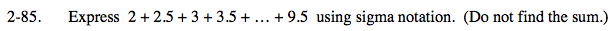### Home > PC > Chapter 2 > Lesson 2.3.2 > Problem2-85

2-85.

Express 2 + 2.5 + 3 + 3.5 + … + 9.5 using sigma notation. (Do not find the sum.) Homework Help ✎How many terms are there?

The terms in this series are linear. The first term is 2, the second term is 2.5, etc. Think about how you could write the equation of a line passing through the points (1, 2) and (2, 2.5).

Use the expression in your answer to Step 2 to write the associated sigma notation.

16

$y=0.5x+1.5$

$\sum_{k=1}^{16}(0.5k+1.5)$